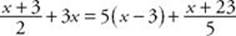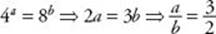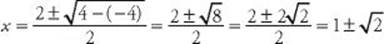﻿ ﻿Exercises - Equations and Inequalities - ALGEBRA - SAT SUBJECT TEST MATH LEVEL 1

## ALGEBRA## CHAPTER 6Equations and Inequalities### Exercises1. If 4x + 13 = 7 – 2x, what is the value of x ?

(A)(B) –3

(C) –1

(D) 1

(E)2. If, what is the value of x?

(A) 3

(B) 5

(C) 7

(D) 9

(E) 11

3. For what values of x is |2x – 3| – 4 < 7?

(A) x < 7

(B) 0 < x < 3

(C) –4 < x < 7

(D) x < 0 or x > 3

(E) x < –4 or x > 7

4. Which of the following is a solution of the equation ?(A) 2

(B) 4

(C) 16

(D) 32

(E) This equation has no solution.

5. If axb = cdx, what is the value of x in terms of a, b, c, and d ?

(A)(B)(C)(D)(E)6. If the sum of two numbers is 13 and the difference of the numbers is 23, what is the product of the numbers?

(A) –90

(B) –23

(C) 0

(D) 18

(E) 36

7. If 4a= 8b, what is the ratio of a to b ?

(A)(B)(C)(D)(E)8. If 4a= 5b, what is the ratio of a to b ?

(A) 0.8

(B) 0.86

(C) 1.08

(D) 1.16

(E) 1.25

9. If r1 and r2 are the two roots of the equation x 2 – 2x – 1 = 0 and if r1 > r2, what is r1r2?

(A)(B) –2

(C) 0

(D)(E) 2

10. If x 2 + 5x + c = 0 has exactly one solution, what is the value of c ?

(A) –6.25

(B) –2.25

(C) 0

(D) 3.75

(E) 6.25

 1. (C) 5. (A) 8. (D) 2. (C) 6. (A) 9. (D) 3. (C) 7. (C) 10. (E) 4. (E)

Solutions

Each of the problems in this set of exercises is typical of a question you could see on a Math 1 test. When you take the model tests in this book and, in particular, when you take the actual Math 1 test, if you get stuck on questions such as these, you do not have to leave them out—you can almost always answer them by using one or more of the strategies discussed in the “Tactics” chapter. The solutions given here do not depend on those strategies; they are the correct mathematical ones.

See Important Tactics for an explanation of the symbol ⇒, which is used in several answer explanations.

1. (C) Add 2x to each side: 6x + 13 = 7. Subtract 13 from each side: 6x = –6. Divide each side by 6: x = –1.

2. (C) Use the six-step method:
(1) Multiply both sides of the equation by 10 to get rid of fractions:

5(x + 3) + 30x = 50(x – 3) + 2(x + 23)

(2) Use the distributive law to get rid of parentheses:

5x + 15 + 30x = 50x – 150 + 2x + 46

(3) Combine like terms:

35x + 15 = 52x – 104

(4) Get the variable onto one side:

15 = 17x – 104

(5) Add 104 to each side:

119 = 17x

(6) Divide by 17:x = 7

3. (C)4. (E). Since by definition a square root cannot be negative, this equation has no solution.

5. (A) Treat a, b, c, and d as constants, and use the six-step method to solve for x:6. (A) Represent the numbers by x and y. Then x + y = 13 and xy = 23. Now add the equations:So. Finally, the product xy = (18)(–5) = –90.

7. (C)Therefore,Alternatively, you could use logarithms as in Solution 8 below.

8. (D)9. (D) Use the quadratic formula to find r1 and r2. Since a = 1, b = –2, and c = –1:Then r1 = 1+and r2 = 1–, and so:10. (E) If a quadratic equation has exactly one solution, then its discriminant is 0. So:﻿